# Fractional inequality, as razvesti fractional inequalities

## Scheme the solution of the fractional inequalities

1. Using the method of intervals

1. Move all members to one side
2. Reduce to a common denominator, always keeping the denominator
2. The use of equivalent transformations
1. Fix odz initial equation
2. Bring to mind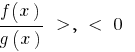and Rozvadov these inequalities for DHS initial or performed the equivalent transformation to introduce a convenient change of variables.
3. Perform background checks on substitution in the original equation

## Equivalent transformation of simple fractional inequalities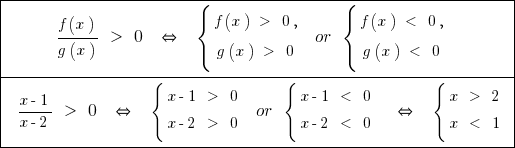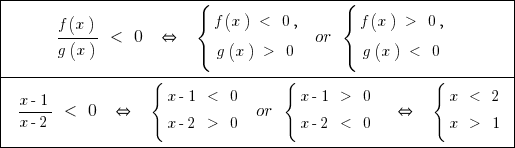## An example of the solution of fractional inequalities method of intervals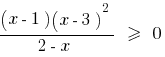1. DHS: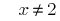2. The zeros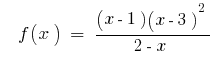inbecause3. Note the zeros at DHS and find the sign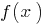in each interval

Answer: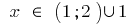orTags:
Chapter:
Versions in other languages:
Share with friends: